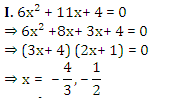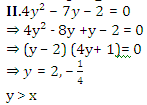Courses

# Quantitative MCQ - 17

## 30 Questions MCQ Test Quantitative Aptitude for Competitive Examinations | Quantitative MCQ - 17

Description
This mock test of Quantitative MCQ - 17 for Quant helps you for every Quant entrance exam. This contains 30 Multiple Choice Questions for Quant Quantitative MCQ - 17 (mcq) to study with solutions a complete question bank. The solved questions answers in this Quantitative MCQ - 17 quiz give you a good mix of easy questions and tough questions. Quant students definitely take this Quantitative MCQ - 17 exercise for a better result in the exam. You can find other Quantitative MCQ - 17 extra questions, long questions & short questions for Quant on EduRev as well by searching above.
QUESTION: 1

### Directions (1-5): What approximate value should come in place of the question mark (?) in the following questions? (10.97)² + (4.03)³ × 3.99 = ?

Solution: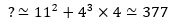QUESTION: 2

### Directions (1-5): What approximate value should come in place of the question mark (?) in the following questions? 12.10% of 935.01 + 1499.98% of 25.95 = ?

Solution: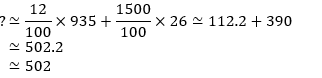QUESTION: 3

### Directions (1-5): What approximate value should come in place of the question mark (?) in the following questions? ∛65×23.93-31.04= ?

Solution: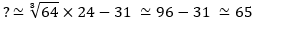QUESTION: 4

Directions (1-5): What approximate value should come in place of the question mark (?) in the following questions?

1624.02 × 3.991 = ?

Solution: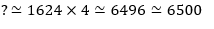QUESTION: 5

Directions (1-5): What approximate value should come in place of the question mark (?) in the following questions?

3018.01 ÷ 2.97 – 841.02 = ?

Solution: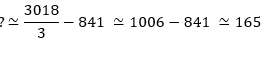QUESTION: 6

Two vessels contain the spirit of 0.5 and 0.75 concentrations. If 2 litres from the first vessel and 3 litres from the second vessel are mixed, then what will be the ratio of the spirit and the water in the resultant solution?

Solution: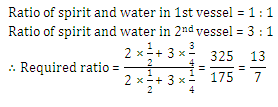QUESTION: 7

In a mixture of 60 litres, the ratio of milk and water is 2 : 1. If the ratio of the milk and water is to be 1 : 2, then the amount of water to be further added must be

Solution: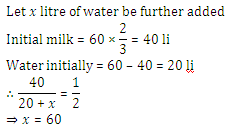QUESTION: 8

A person has a chemical of Rs. 25 per litre. In what ratio should water be mixed in that chemical so that after selling the mixture at Rs. 20 per litre he may get a profit of 25%?

Solution: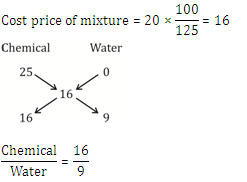QUESTION: 9

How many kg of sugar costing Rs. 5.75 per kg should be mixed with 75 kg of cheaper sugar costing Rs. 4.50 per kg so that the mixture is worth Rs. 5.50 kg?

Solution: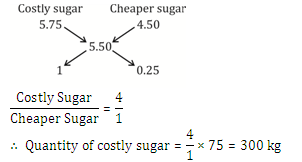QUESTION: 10

In a zumba class, the average age of all the members was 43.5 years. 10 members left the class and 6 new members joined. If the average age increased by 2 years and the total age decreased by 110, what was the number of members in the class initially?

Solution: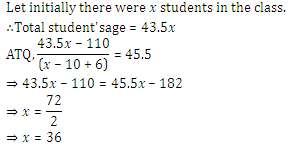QUESTION: 11

There are 5 consecutive odd numbers. If the difference between the square of the average of first two odd numbers and the square of the average of last two odd numbers is 588, what is the smallest odd numbers?

Solution: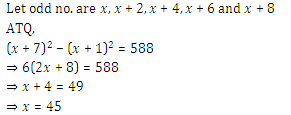QUESTION: 12

Directions (Q12,13): What should come in place of question mark (?) in following simplification problems?

0.009 + 0.001 ÷ 10 + 0.003 = ?

Solution: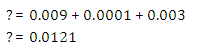QUESTION: 13

Directions (Q12,13): What should come in place of question mark (?) in following simplification problems?

48% of 950 – 46% of 840 = ?

Solution: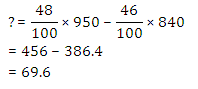QUESTION: 14

Directions (14-18): Study the pie-charts carefully to answer the questions that follow:

Percentage of employees and women working in different Departments of an Organisation

Percentage of employees working in different department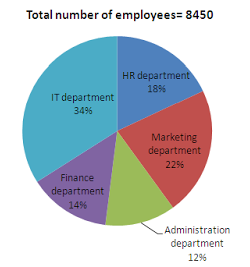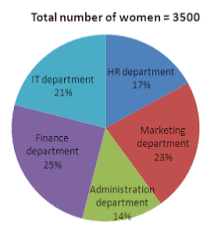Which department has the lowest number of Men working in it?

Solution:

From pie chart we can say that two different departments i.e. administration and finance have number of men employee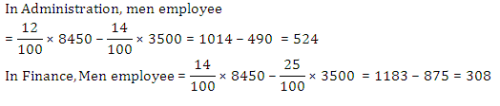QUESTION: 15

Directions (14-18): Study the pie-charts carefully to answer the questions that follow:

Percentage of employees and women working in different Departments of an Organisation

Percentage of employees working in different departmentWhat is the total number of men working in HR, Marketing and IT departments together in the organisation?

Solution: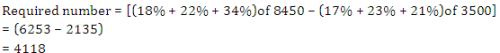QUESTION: 16

Directions (14-18): Study the pie-charts carefully to answer the questions that follow:

Percentage of employees and women working in different Departments of an Organisation

Percentage of employees working in different departmentWhich is the respective ratio of the number of women working in Administration department to the total number of employees working in that department?

Solution: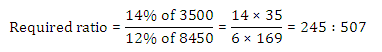QUESTION: 17

Directions (14-18): Study the pie-charts carefully to answer the questions that follow:

Percentage of employees and women working in different Departments of an Organisation

Percentage of employees working in different departmentThe number of women working in the Finance and IT department together form what percent of total number of employees in the organisation? (rounded off to two digits after decimal)

Solution: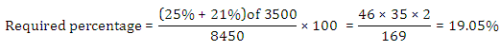QUESTION: 18

Directions (14-18): Study the pie-charts carefully to answer the questions that follow:

Percentage of employees and women working in different Departments of an Organisation

Percentage of employees working in different departmentWhat is the number of men working in the HR department?

Solution: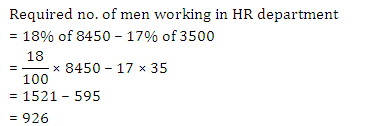QUESTION: 19

The wheat sold by a grocer contained 10% low quality wheat. What quantity of good quantity wheat should be added to 150 kg of wheat so that the percentage of low-quality wheat becomes 5%?

Solution: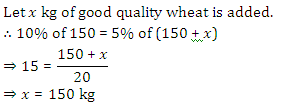QUESTION: 20

Two pipes can fill a cistern in 14 and 16 hours respectively. The pipes are opened simultaneously and it is found that due to leakage in the bottom of the cistern, it takes 32 minutes extra for the cistern to be filled up. When the cistern is full, in what time will the leak empty it?

Solution: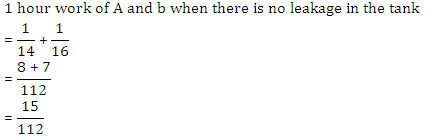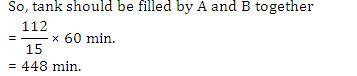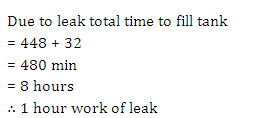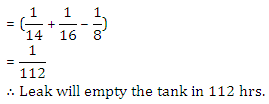QUESTION: 21

The ratio between the speed of a train and a car is 18 : 3 respectively. Also, a bus covered a distance of 480 kms in 12 hours. The speed of the bus is five-ninths the speed of the train. How much distance will the car cover in 5 hours?

Solution: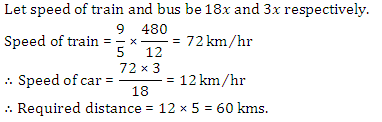QUESTION: 22

Train-A crosses a pole in 25 seconds and another Train-B crosses as pole in 1 minute and 15 seconds. The Length of the train-A is half the length of the train-B. What is the respective ratio between the speed of the train-A and the train-B?

Solution: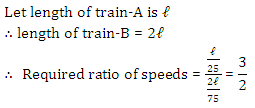QUESTION: 23

Directions (23-25): What value should come in the place of question mark (?) in the following questions?

3.6 + 36.6 + 3.66 + 0.36 + 3.0 = ?

Solution: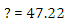QUESTION: 24

Directions (23-25): What value should come in the place of question mark (?) in the following questions?

23 × 45 ÷ 15 = ?

Solution: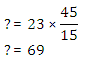QUESTION: 25

Directions (23-25): What value should come in the place of question mark (?) in the following questions?

83% of 2350 = ?

Solution: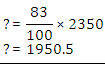QUESTION: 26

Directions (6-10): In the given question, two equations numbered I and II are given. You have to solve both the equations and Give answer:

I. 2x² - 7x+ 3 = 0

II. 2y² - 7y + 6 = 0

Solution: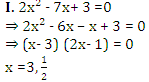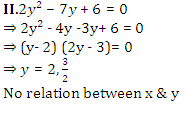QUESTION: 27

Directions (6-10): In the given question, two equations numbered I and II are given. You have to solve both the equations and Give answer:

I. 4x² + 16x +15 = 0

II. 2y² + 3y + 1 = 0

Solution: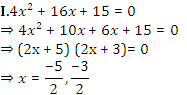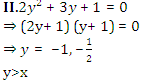QUESTION: 28

Directions (6-10): In the given question, two equations numbered I and II are given. You have to solve both the equations and Give answer:

I. 9x² - 45x + 56 = 0

II. 4y² - 17y + 18 = 0

Solution: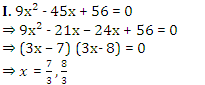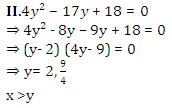QUESTION: 29

Directions (6-10): In the given question, two equations numbered I and II are given. You have to solve both the equations and Give answer:

I. 2x² + 11x + 14 = 0

II. 2y² + 15y + 28 = 0

Solution: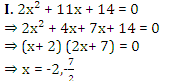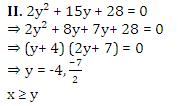QUESTION: 30

Directions (6-10): In the given question, two equations numbered I and II are given. You have to solve both the equations and Give answer:

I. 6x² + 11x + 4 = 0

II. 4y² - 7y – 2 = 0

Solution: